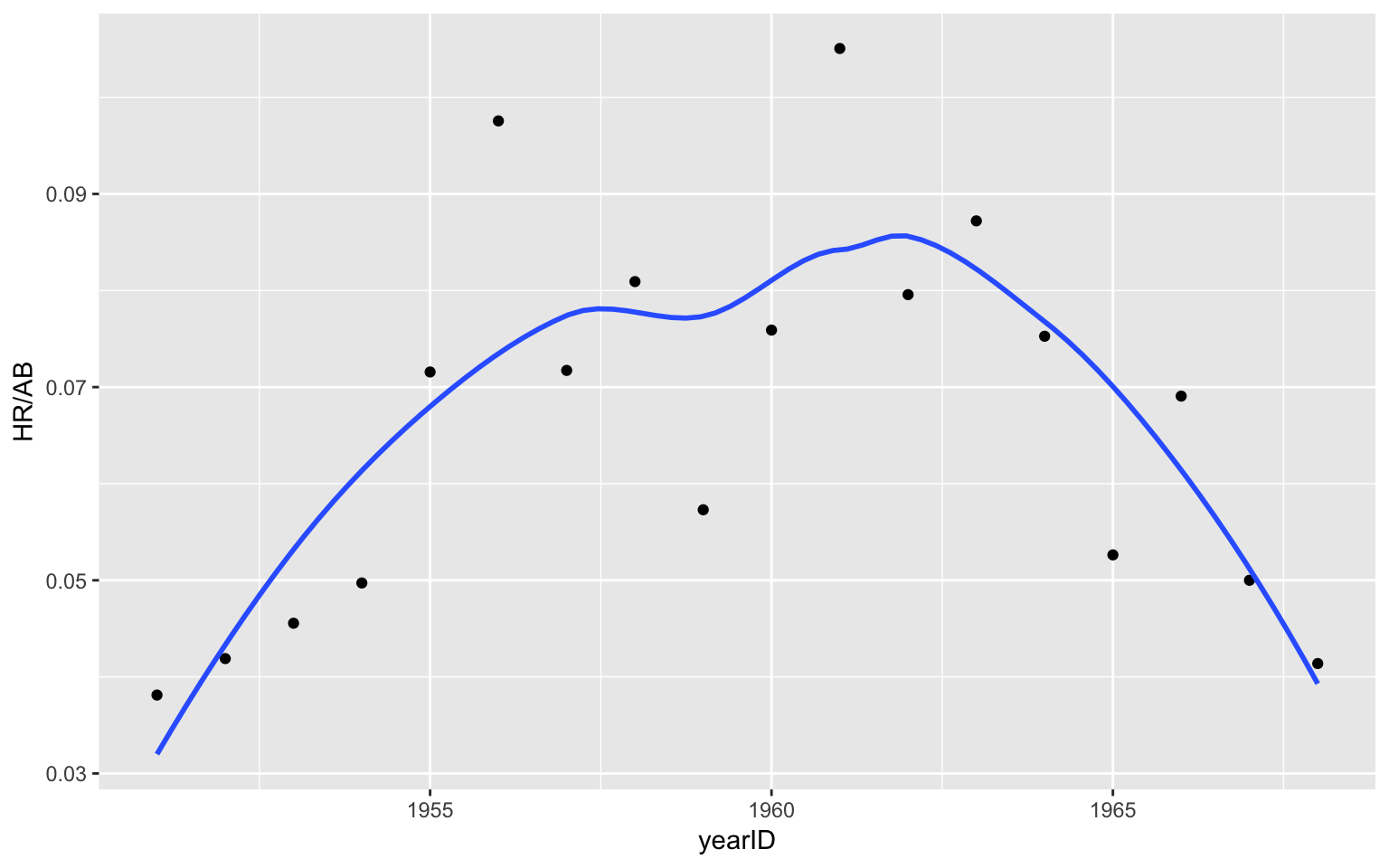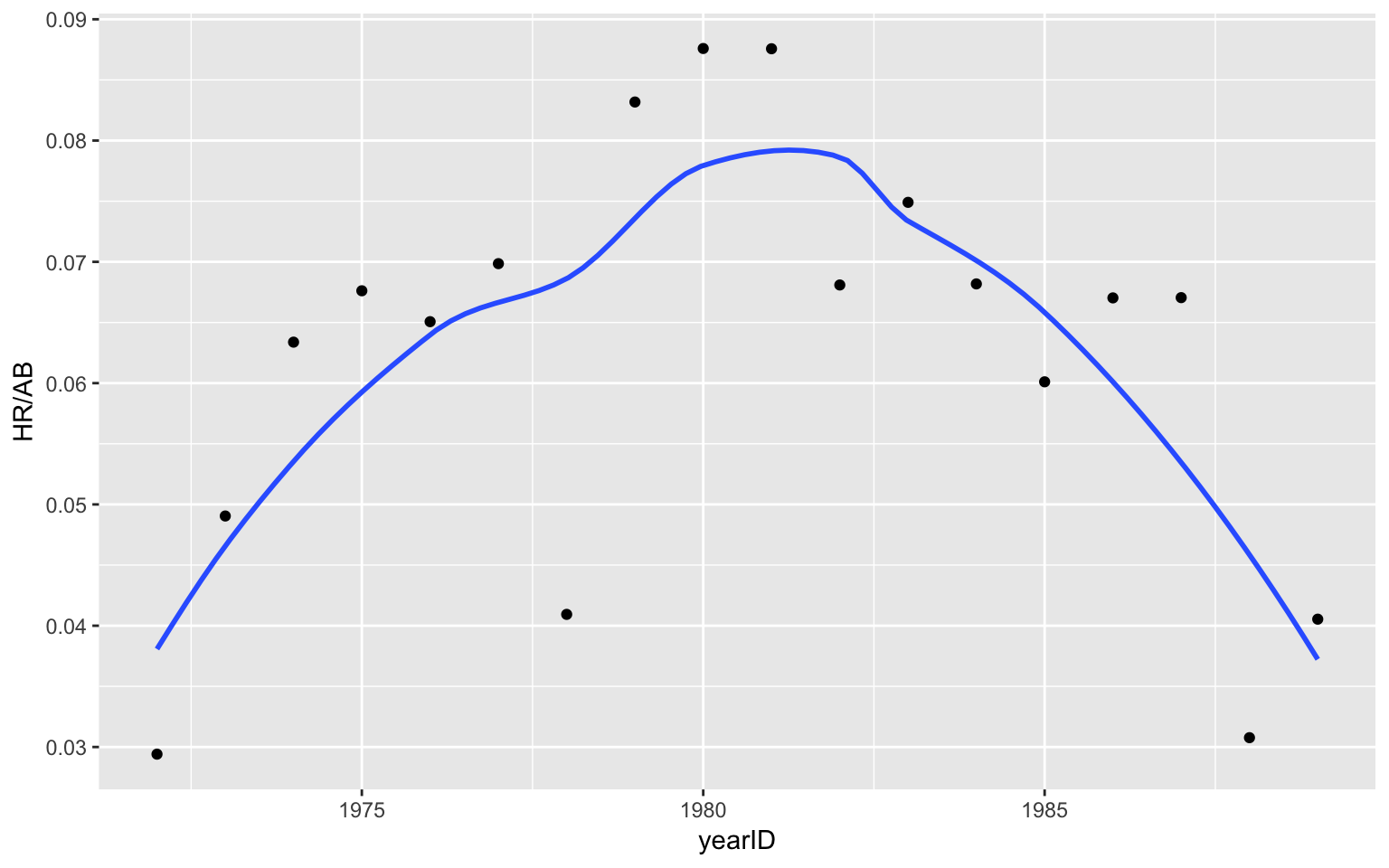### Plotting a Career Trajectory

#### Writing a Plot Trajectory Function

Here is a function `plot_hr_trajectory` that will graph a specific player’s home run trajectory. It uses three packages: `Lahman` contains the season-to-season data, `dplyr` helps with data management, `stringr` helps with one string operation, and `ggplot2` does the graphing.

Here is some insight how `plot_hr_trajectory` works:

1. The input is the player’s full name in quotes.

2. Using the `Master` data frame in the `Lahman` package, I find the `playerID` and birth information for that player.

3. From the `Batting` data frame of hitting data, I collect `HR`, `AB` for all seasons of the player’s career.

4. I find the `Age` variable by first finding the player’s birthyear, adjusting the birthyear depending on the birthmonth, and then defining `Age`.

5. I use `ggplot2` to construct a scatterplot and smoothing curve for the home run rate `HR` / `AB`.

``````plot_hr_trajectory <- function(playername){
require(Lahman)
require(dplyr)
require(stringr)
require(ggplot2)
names <- unlist(str_split(playername, " "))
info <- filter(Master, nameLast==names,
nameFirst==names)

bdata <- filter(Batting, playerID==info\$playerID)
bdata <- mutate(bdata,
birthyear = ifelse(info\$birthMonth >= 7,
info\$birthYear + 1, info\$birthYear),
Age = yearID - birthyear)

ggplot(bdata, aes(yearID, HR / AB)) +
geom_point() +
geom_smooth(method="loess", se=FALSE)
}``````

#### Plotting Two Trajectories

I illustrate using this function for two players. Note that I am saving the `ggplot2` plotting object in a variable. By just typing the variable name, I see the graph.

``````p1 <- plot_hr_trajectory("Mickey Mantle")
p1````````````p2 <- plot_hr_trajectory("Mike Schmidt")
p2``````#### Comparing Trajectories

The `ggplot2` object contains the plotting data. So I combine the data from the two earlier plotting objects to construct a graph that compares the two trajectories.

``````ggplot(rbind(p1\$data, p2\$data), aes(Age, HR / AB)) +
geom_point() +
geom_smooth(method="loess", se=FALSE) +
facet_wrap(~ playerID, ncol=1)``````